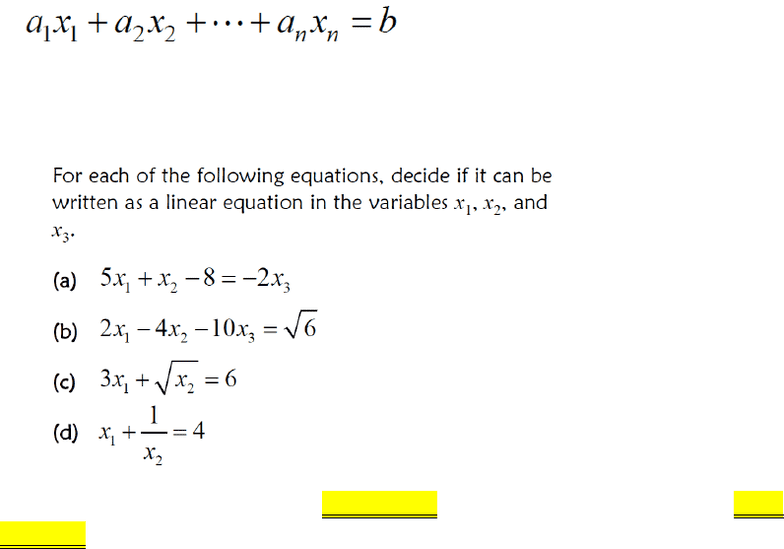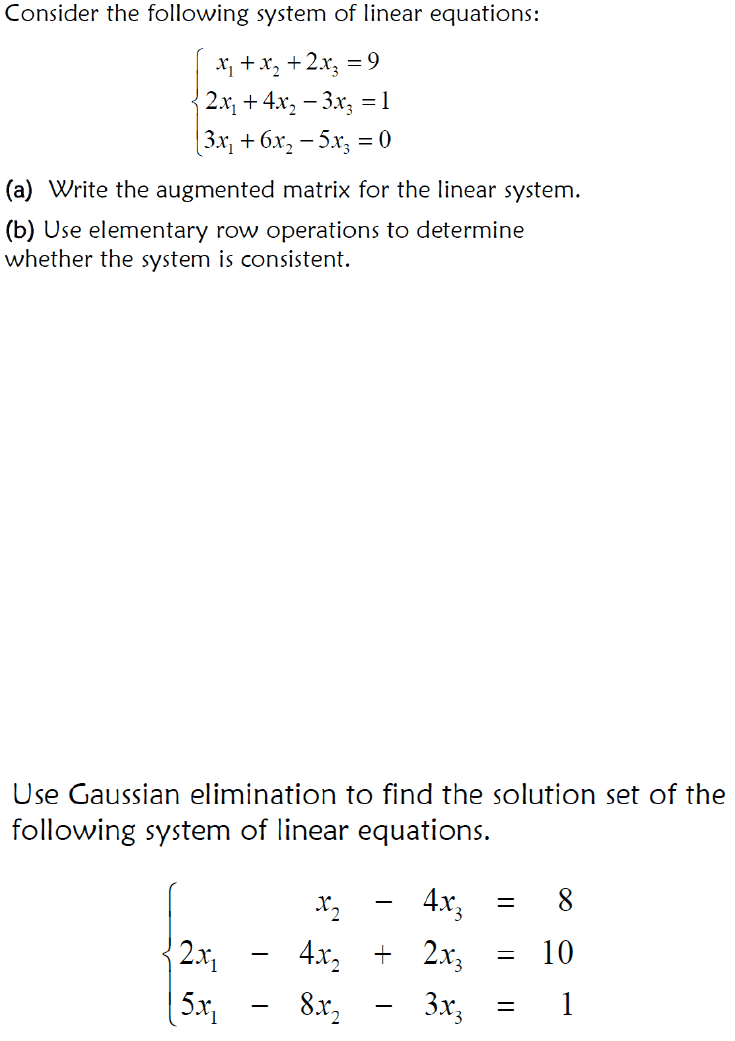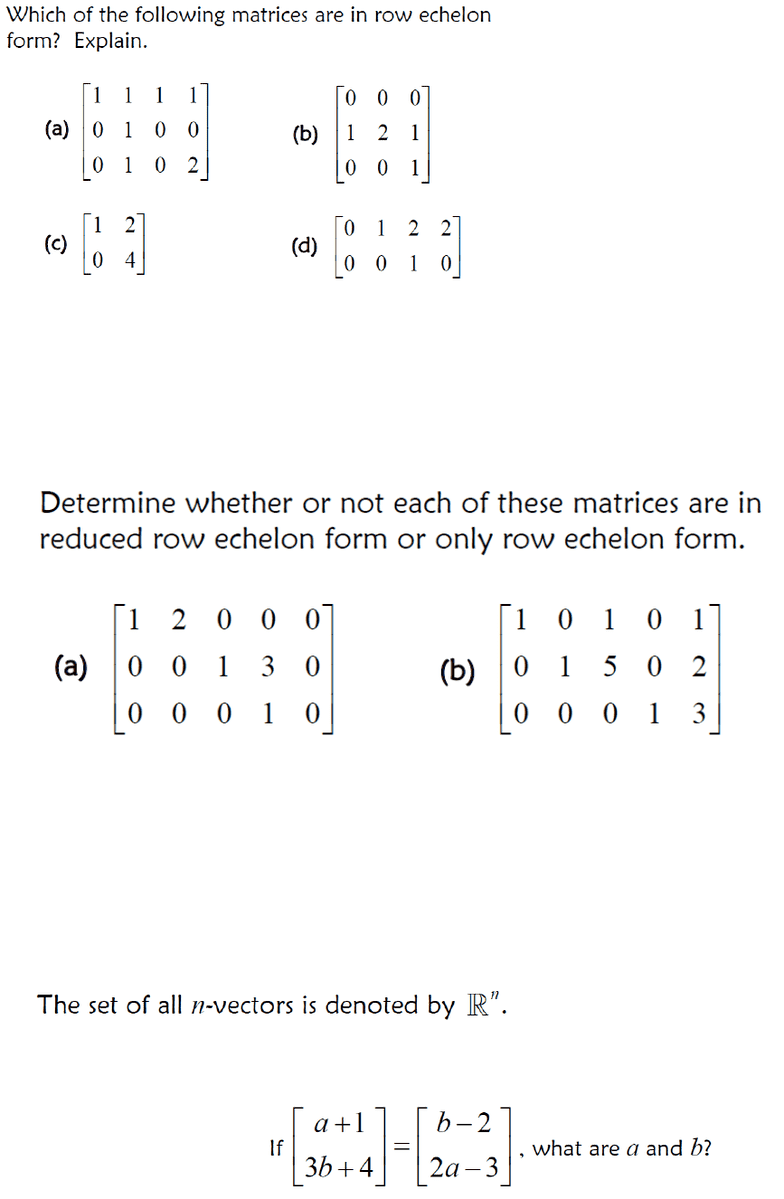Study Guides (380,000)
CA (150,000)
Carleton (5,000)
MATH (200)
Final

# MATH 1119 Final: Math 1119 Final Review

Department
Mathematics
Course Code
MATH 1119
Professor
Gang Li
Study Guide
Final

This preview shows pages 1-3. to view the full 14 pages of the document.Section 1.1 Systems of Linear Equations
1. A Linear equation is an equation that can be write in this form:
“a” and “b” are constants, the power of “x” is 1.
Exercise: (from slide exercise 1)
2. A linear system is a collection of one or more linear equations involving the same
variables.
3. If a system of linear equation is
a) Consistent : at least one solution ( one solution or infinitely many
solutions)
b) Inconsistent: no solution
4. Equivalent System of Linear Equations:
Two systems of linear equations are equivalent if they have the exact same
solution set.
5. Row equivalent matrices:
If a series of row operation are applied to a matrix to obtain a new matrix, the
two matrices are said to be row equivalent.
Tips: when you apply a row operation, always remember to write the notations!!! That
worth 1 mark.
Exercise: (from slide exercise 9)

Only pages 1-3 are available for preview. Some parts have been intentionally blurred.Section 1.2 Row reduction and Echelon Form
1. Gaussian Elimination
Step 1: Find the left-most non-zero column and select a non-zero entry in that column.
Interchange rows if necessary so that this entry is in the first row.
Step 2: Divide the top row by the non-zero entry from Step 1, there should now be a 1 in the
top left entry. This is called a “leading one” or a “pivot”.
Step 3: In that same column, change all of the entries beneath the pivot to zeros by adding or
subtracting multiples of the top row.
Step 4: Cover up the top row and repeat Steps 1 through 3.
Exercise (from slides exercise 2)
2. Row Echelon Form
a) The first non-zero entry in each row is a 1 (leading one)
b) Each leading one is to the right of the leading one in the row above it
c) Any rows containing only zeroes are grouped at the bottom of the matrix
Exercise (from slide, exercise 3)

Only pages 1-3 are available for preview. Some parts have been intentionally blurred.3. Reduced Row Echelon Form
a. The matrix is in row echelon form.
b. Each leading one is the only non-zero entry in its column.
Exercise (from slide, exercise 6)
4. No free variable: unique solution or no solution
At least on free variable: infinitely many solutions
Section 1.3 Vector Equations
1. Vector is a matrix with only one column
2. A vector with n rows or entries is also known as an n-vector.
3.
4. Equality of vector: Two vectors u and v are said to be equal, that is u = v, if and only if
they have the same number of entries and their corresponding entries are equal.
Exercise (from slide, exercise 1)
###### You're Reading a Preview

Unlock to view full version